# Difference between revisions of "Module MacroAlgae"

## Overview

Module MacroAlgae is a zero-dimensional biogeochemical model and was designed to compute the main biogeochemical processes related with macroalgae in aquatic environments. This module simulates two types of macroalgae:

- Attached macroalgae - macroalgae attached to the bottom of the water column

- Drifting macroalgae - macroalgae dettached from the bottom drifting in the water column


The evolution of the two macroalgae types is computed in the exactly same way (although different parameters are allowed for each type). The main differences relate to the fact that attached macroalgae are not transported by flow and drifting macroalgae are. On top of the main primary production limiting factors (e.g. light, nutrients, temperature), attached macroalgae growth is also limited by salinity (optionally), by suspended particulate matter deposition fluxes and also by shear stress near the bottom, which dettaches these macroalgae which become drifting macroalgae. Drifting macroalgae do not have this type of limitation (SPM deposition flux and obviously bottom shear stress) and when they reach the shore the only process computed is an increased mortality rate.

This module is independent of the pelagic and benthic biogeochemical model being used to compute other biogeochemical processes, thus it can be coupled with Module WaterQuality, Module CEQUALW2 or Module Life, or even Module Benthos.

One feature of this macroalgae model is that it is fully coupled with the 3D transport model, as macroalgae, although attached in the bottom, can influence water properties in the all water column and not only to the grid cell near the bottom (e.g. light attenuation, nutrients uptake, etc). This is the case when the macroalgae height is higher than the grid cell height. Basically, an occupation ratio is computed for each grid cell, based on the bottom distribution and macroalgae height.

## Equations

As mentioned before, attached macroalgae evolution, in terms of biogeochemical processes, is computed exactly the same way as drifting macroalgae, and can be written as: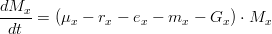$\frac{dM _x}{dt} = (\mu _x - r_x - e_x - m_x - G_x) \cdot M _x$


where,

*$x$ is the type of macroalgae (attached or drifting)
*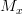$M _x$ is the macroalgae distribution
*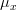$\mu _x$ is the gross production rate (in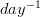$day^{-1}$)
*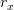$r_x$ is the respiration rate (in$day^{-1}$)
*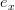$e_x$ is the excretions rate (in$day^{-1}$)
*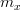$m_x$ is the natural mortality rate (in$day^{-1}$)
*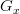$G_x$ is the lost chain grazing rate (in$day^{-1}$)


### Gross production rate

The gross production rate$\mu _x$ is given by: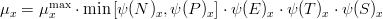$\mu _x = \mu^{\max }_x \cdot \min \left[{\psi (N)_x ,\psi (P)_x} \right]\cdot \psi (E)_x \cdot \psi (T)_x \cdot \psi (S)_x$


where,

*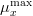$\mu^{\max }_x$ is the macroalgae maximum growth rate (in$day^{-1}$)
*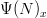$\Psi (N)_x$ is the nitrogen limiting factor
*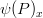$\psi (P)_x$ is the phosphorus limiting factor
*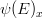$\psi (E)_x$ is the light limiting factor
*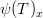$\psi (T)_x$ is the temperature limiting factor
*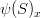$\psi (S)_x$ is the salinity limiting factor


### Limiting factors

#### Nutrients

The nitrogen limiting factor can be computed as: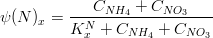$\psi (N)_x = \frac{{C_{NH_4} + C_{NO_3}}} {{K^N_x + C_{NH_4} + C_{NO_3}}}$


where,

*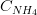$C_{NH_4}$ is the ammonia concentration in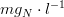$mg_N \cdot l^{-1}$
*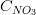$C_{NO_3}$ is the nitrate concentration in$mg_N \cdot l^{-1}$
*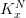$K^N_x$ is the nitrogen half-saturation constant in$mg_N \cdot l^{-1}$


The phosphorus limiting factor can be computed as: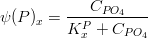$\psi (P)_x = \frac{{C_{PO_4}}} {{K^P_x + C_{PO_4}}}$


where,

*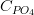$C_{PO_4}$ is the inorganic phosphorus concentration in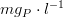$mg_P \cdot l^{-1}$
*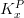$K^P_x$ is the phosphorus half-saturation constant in$mg_P \cdot l^{-1}$


#### Temperature

Macroalgae's temperature limiting factor is given by: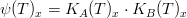$\psi (T)_x = K_A (T)_x \cdot K_B (T)_x$


where,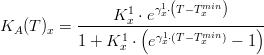$K_A (T)_x = \frac {K^1_x \cdot e^{\gamma^1_x \cdot \left( {T - T^{min}_x} \right)}} {{1 + K^1_x \cdot \left( {e^{\gamma^1_x \cdot \left({T - T^{min}_x } \right)} - 1} \right)}}$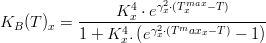$K_B (T)_x = \frac{K^4_x \cdot e^{\gamma^2_x \cdot \left( {T^{max}_x - T} \right)}} {{1 + K^4_x .\left( {e^{\gamma^2_x \cdot \left( {T^max_x - T} \right)} - 1} \right)}}$


### Respiration rate

The macroalgae respiration rate is given by: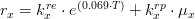$r_x = k^{re}_x \cdot e^{(0.069 \cdot T)} + k^{rp}_x \cdot \mu_x$


where,

*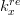$k^{re}_x$ is the macroalgae endogenous respiration (in$day^{-1}$)
*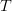$T$ is the water temperature (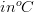$in ^o C$)
*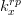$k^{rp}_x$ is the macroalgae photo respiration rate (in$day^{-1}$)


### Excretion rate

The macroalgae excretion rate is given by: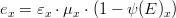$e_x = \varepsilon_x \cdot \mu_x \cdot (1 - \psi (E)_x )$


where,

*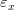$\varepsilon_x$ is the reference excretion rate (in$day^{-1}$)


### Natural mortality rate

The macroalgae natural mortality rate is given by: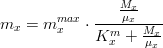$m_x = m^{max}_x \cdot \frac{{\frac{{M_x}} {{\mu _x}}}} {{K^m_x + \frac{{M_x}} {{\mu_x}}}}$


where,

*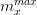$m^{max}_x$ is the maximum natural mortality rate (in$day^{-1}$)
*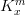$K^m_x$ is the half-saturation constant


## Numerical scheme

Module MacroAlgae solves the differential equations described above using an Euler forward scheme (explicit method), which was used in order to make the code organization as simple as possible. When using this numerical method there are, nevertheless, some restraints in terms of temporal discretization, which can cause numerical instabilities that can be solved, at some extent, by using a smaller time step.

Only for a specific calculation (drifting macroalgae mortality when reaching the shore), an Euler backward scheme (implicit method) is used to insure numerical stability.

Module MacroAlgae has an independent time step in relation to the model’s main time step. Normally the time step to integrate the differential equations describing these kind of biogeochemical reactions is much higher than the time step used to solve hydrodynamic and transport equations, and as the set of equations in this module is quite simple, there is no significant increase in terms of computational costs when using a smaller time step.

## User guide

### Waterproperties data file options

In order to activate this module, specify in Module WaterProperties input data file, in each property involved in macroalgae processes the following keyword:

MACROALGAE             : 1


Here is the property list which has to be with the macroalgae processes keyword activated:

macroalgae
drifting macroalgae
oxygen
ammonia*
nitrate*
dissolved non-refractory organic nitrogen*
particulate organic nitrogen*
inorganic phosphorus**
dissolved non-refractory organic phosphorus**
particulate organic phosphorus**


*if computing the nitrogen cycle

**if computing the phosphorus cycle

The following step, still in Module WaterProperties input data file, in the header section, the following keywords:

MACROALGAE_MASS       : 0.001        !macroalgae bottom distribution in gC/m2
MACROALGAE_HEIGHT     : 0.25         !macroalgae reference height in meters


Ultimately one must parameterize the influence of macroalgae in light extincion. Thus, in the macroalgae property block the following keywords must be defined:

LIGHT_EXTINCTION      : 1            !activates macroalgae influence on light extinction
EXTINCTION_PARAMETER  : 0.01         !defines the extinction parameter for macroalgae


Remember that in property macroalgae advection-diffusion should be off:

ADVECTION_DIFFUSION   : 0


Property drifting macroalgae advection-diffusion should be on.

### Macroalgae data file options

Then, activate Module MacroAlgae in MOHID GUI as described here, which enables to edit the MacroAlgae input data file. In this file, data is stored and organized in keywords and blocks. The main keywords are:

Keyword            Default value       Description
DT                  : 3600]          !Time step compute biogeochemical processes
PELAGIC_MODEL       : WaterQuality   !Defines which pelagic biogeochemical model is coupled
NITROGEN            : 0              !Compute the nitrogen cycle
PHOSPHORUS          : 0              !Compute the phosphorus cycle


For each macroalgae type a block must be specified:

Attached macroalgae - <begin_macroalgae>         ... <end_macroalgae>
Drifting macroalgae - <begin_driftingmacroalgae> ... <end_driftingmacroalgae>


Inside each block specific parameters are defined:

Keyword           Default value      Description
GROWMAX             : 0.4          !maximum growth rate
TOPTMIN             : 20.          !optimum minimum temperature for growth
TOPTMAX             : 25.          !optimum maximum temperature for growth
TMIN                : 5            !minimum temperature for growth
TMAX                : 40.          !maximum temperature for growth
TCONST1             : 0.05         !constant to control temperature response curve shape
TCONST2             : 0.98         !constant to control temperature response curve shape
TCONST3             : 0.98         !constant to control temperature response curve shape
TCONST4             : 0.02         !constant to control temperature response curve shape
PHOTOIN             : 90.          !optimum radiation value
ENDREPC             : 0.009        !endogenous respiration rate
PHOTORES            : 0.018        !photorespiration rate
EXCRCONS            : 0.008        !excretion rate
MORTMAX             : 0.003        !natural mortality rate
MORTCON             : 0.03         !mortality half saturation constant
GRAZCONS            : 0.00008      !grazing rate over macroalgae
SOLEXCR             : 0.25         !fraction of soluble inorganic material excreted by macroalgae
DISSDON             : 0.25         !fraction of dissolved organic material excreted by macroalgae
NSATCONS            : 0.065        !nitrogen half-saturation constant for macroalgae
PSATCONS            : 0.001        !phosphorus half-saturation constant for macroalgae
RATIONC             : 0.18         !macroalgae nitrogen/carbon ratio
RATIOPC             : 0.024        !macroalgae phosphorus/carbon ratio
MACROALGAE_MINCONC  : 1e-12        !minimum residual value for macroalgae abundance
MIN_OXYGEN          : 1e-8         !minimum oxygen concentration for macroalgae growth
DEPLIM              : 5e-6         !maximum SPM deposition flux for macroalgae growth(kg m-2 s-1)*
EROCRITSS           : 0.1          !critical shear stress to occur macroalgae dettachment (in Pa)*
SALT_EFFECT         : 0            !include salinity limitation on macroalgae growth
SALTOPT             : 20           !macroalgae optimum salinity for growth
SALTCRIT            : 5.           !macroalgae critical salinity limit growth
SALTMIN             : 0.           !macroalgae minimum salinity for growth
SALTMAX             : 45.          !macroalgae maximum salinity for growth
BEACHED_MORT_RATE   : 0.01         !beached drifting macroalgae mortality rate**


*only for attached macroalgae

**only for drifting macroalgae

## Performing outputs of biogeochemical rates

In order to perform outputs of biogeochemical rates and other parameters of the MacroAlgae model, you can define in the WaterProperties input data file the following options.

BOXFLUXES          : ..\..\GeneralData\Boxes.dat     !path to a boxes data file


The same keyword, referencing the same boxes data file must be defined in the Hydrodynamic input data file. This insures the output of the water.BXM file, which contains the spatial integration of the water volumes into the previously boxes defined.

Also at least one water property needs to have the following keyword activated:

BOX_TIME_SERIE     : 1


Next step is to define the rates which the user wants to analyse. This is done similarly to the other biogeochemical modules. All exchange rates are available for output (e.g. macroalgae to ammonia, ammonia to macroalgae, oxygen to macroalgae, macroalgae to oxygen, etc...)

This can be done by defining in WaterProperties input data file, a block as the one presented below:

<beginwqrate>
NAME                      : macroalgae ammonia uptake
DESCRIPTION               : what goes from ammonia to macroalgae
FIRSTPROP                 : ammonia
SECONDPROP                : macroalgae
MODEL                     : MacroAlgae
<endwqrate>

<beginwqrate>
NAME                      : macroalgae ammonia excretions
DESCRIPTION               : what goes from macroalgae to ammonia
FIRSTPROP                 : macroalgae
SECONDPROP                : ammonia
MODEL                     : MacroAlgae
<endwqrate>


One can also analyse other parameters such as:

• macroalgae gross production
• macroalgae temperature limiting factor
• macroalgae light limiting factor
• macroalgae nutrients limiting factor
• macroalgae nitrogen limiting factor
• macroalgae phosphorus limiting factor
• macroalgae salinity limiting factor

In these cases, in the <beginwqrate>...<endwqrate> block, the FIRSTPROP keyword relates to the parameter to be analysed and the SECONDPROP relates to the type of macroalgae. For example, if one wishes to analyse the gross production of attached macroalgae and drifting macroalgae, the data file should look like this:

<beginwqrate>
NAME                      : macroalgae gross production
DESCRIPTION               : macroalgae gross production
FIRSTPROP                 : grossprod
SECONDPROP                : macroalgae
MODEL                     : MacroAlgae
<endwqrate>

<beginwqrate>
NAME                      : macroalgae gross production
DESCRIPTION               : macroalgae gross production
FIRSTPROP                 : grossprod
SECONDPROP                : drifting macroalgae
MODEL                     : MacroAlgae
<endwqrate>


In the end a time series file with an *.BXM extension and with the name of the rate (set by keyword 'NAME'), is written.